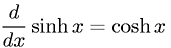Equations > Calculus > Differentiation/Differentals > Derivative of Hyperbolic Sine

### Derivative of Hyperbolic SineLatex Code:

MathML Code:

 $\fracd\mathrm{dx}\mathrm{sinh}x=\mathrm{cosh}x$

MathType 5.0: